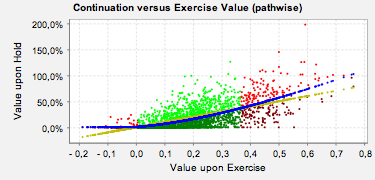# Bermudan (Equity) Option (under lognormal model)

## Java Applet

Alternatively you may launch this applet using Java WebStart. Minimum requirements: Java 1.6. Tested with Firefox and Safari. Source code for the underlying library is available at finmath.net.

IMPORTANT: The applet is currently self-signed. Hence it will no longer run under the Java security level "high", which has become default. In order to run the applet, you need to add the domain http://christian-fries.de to the Exception Site List. To edit the Exception Site List open the Java control panel, select the Security tab, then press Edit Site List.

## Description

This tools illustrated Bermudan option pricing using Monte Carlo simulation.

It serves as a companion to Chapter 15 of Mathematical Finance.

Upon each exercise date T1, T2,...,Tn the holder of the Bermudan has the right to either exercise and receive Ni * (S(Ti) - Ki) or receive a shorter Bermudan option on the remaining exercises Tj > Ti. The last option pays either Nn * (S(Tn) - Kn) or nothing. Setting notional negative will give you a put instead of a call.

### Evaluation Methods

Various methods to estimate the exercise boundary are implemented:

Regression
The method also known as Longstaff-Schwartz where the exercise boundary is estimated by a linear regression on some basis functions.
Binning
The definition space of the predictor variable (here the exercise value) is split into intervals (bins) on which a conditional expectation is calculated.
Threshold optimization
A root finder optimizes a scalar threshold used in a specific exercise criteria (e.g. a linear function of the intrinsic value).
Perfect foresight
The single value given by the future evolution of the path is used as "estimate" for the expectation. This gives a foresight bias an thus a wrong price (the strategy is super optimal).
Analytic
For an Bermudan with only two exercise date and a simple Black-Scholes type model the optimal exercise can be calculated analytically (using Black-Scholes Formula upon exercise).

### Exercise BoundaryFigure 1: Example of the estimation of the exercise boundary.

The exercise boundary is visualized in a scatter plot, see Figure 1. The picture shows the situation at a specific exercise date. Each dot represents the value upon hold depending on the value upon exercise for a specific simulation path. The conditional expectation is shown as a function of the value upon exercise (blue line). The exercise region (green) is defined as the paths having exercise values (yellow line) above the expected value upon hold (blue line).